# imcolordiff

Color difference based on CIE94 or CIE2000 standard

Since R2020b

## Syntax

``dE = imcolordiff(I1,I2)``
``dE = imcolordiff(I1,I2,Name=Value)``

## Description

example

````dE = imcolordiff(I1,I2)` calculates the color difference between two RGB images or color maps using the CIE94 standard.```

example

````dE = imcolordiff(I1,I2,Name=Value)` specifies additional aspects of the computation, such as the input color space and the CIE standard, using one or more name-value arguments.```

## Examples

collapse all

Read a color image into the workspace.

```I1 = imread('peppers.png'); imshow(I1)```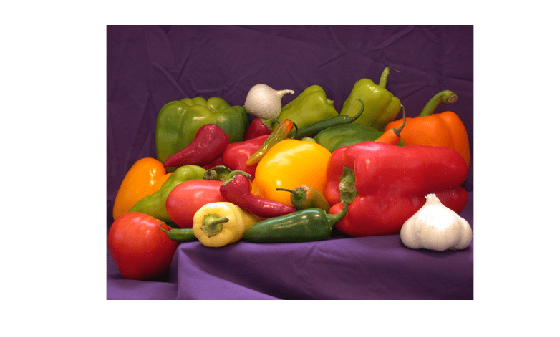Alter the local color contrast in the image.

```I2 = localcontrast(I1); imshow(I2)```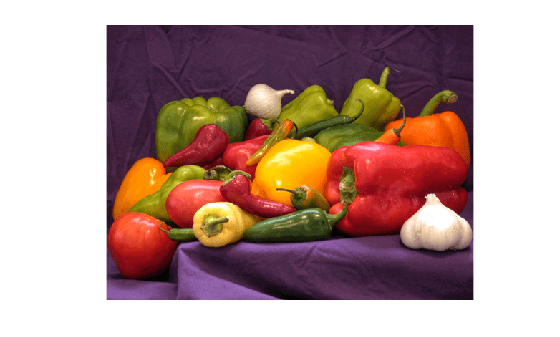Calculate the color difference of the images using the default color standard, CIE94.

`dE = imcolordiff(I1,I2);`

Display the color difference as an image. Scale the display range to use the full range of pixel values in `dE`.

`imshow(dE,[])`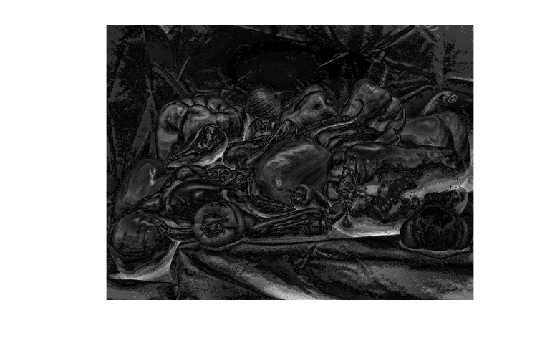Read and display an image of tissue stained with hemotoxylin and eosin (H&E).

```he = imread('hestain.png'); imshow(he)```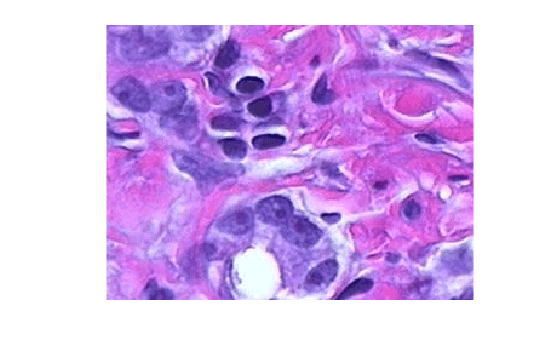Convert the image to the L*a*b* color space.

`lab = rgb2lab(he);`

Make a copy of the image, then increase the signal of the a* channel. Red tones in the image become more saturated while the image overall brightness and the blue tones are unchanged.

```lab2 = lab; scaleFactor = 1.1; lab2(:,:,2) = scaleFactor*lab(:,:,2);```

Calculate the color difference of the original and enhanced image in the L*a*b* color space.

`dE = imcolordiff(lab,lab2,'isInputLab',true);`

Display the color difference as an image. Scale the display range to match the range of pixel values in `dE`. Bright regions indicate the greatest color difference and correspond with the pink regions of tissue.

`imshow(dE,[])`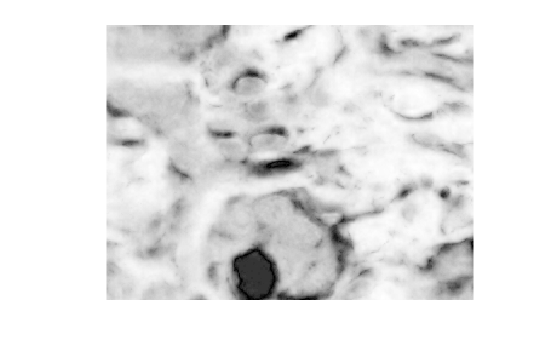Specify two RGB color values.

```pureRed = uint8([255,0,0]); darkRed = uint8([255,10,50]);```

Calculate the color difference of the colors using the CIEDE2000 standard.

`dE = imcolordiff(pureRed,darkRed,"Standard","CIEDE2000")`
```dE = single 7.4449 ```

Read and display an RGB image of fabric.

```fabric = imread('fabric.png'); imshow(fabric)```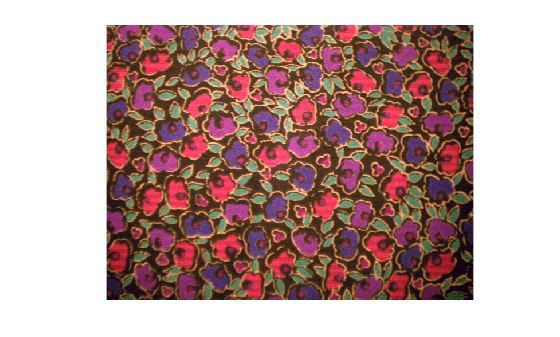Simulate a second image of fabric by altering the local color contrast in the image.

```fabric2 = localcontrast(fabric); imshow(fabric2)```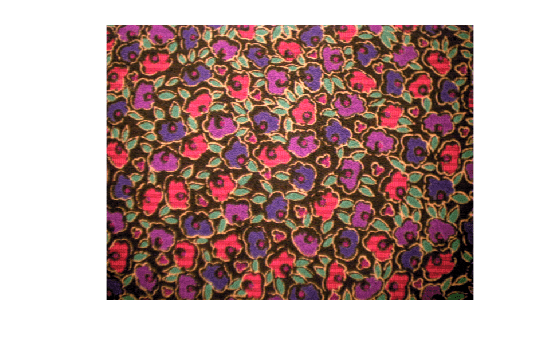Calculate the color difference of the two images using the CIEDE2000 standard. Specify a luminance coefficient and K1 and K2 weighting factors appropriate for textiles.

```dE = imcolordiff(fabric,fabric2,'Standard','CIEDE2000', ... 'kL',2,'K1',0.048,'K2',0.014);```

Display the color difference. Scale the display range to the full range of pixel values in `dE`.

`imshow(dE,[])`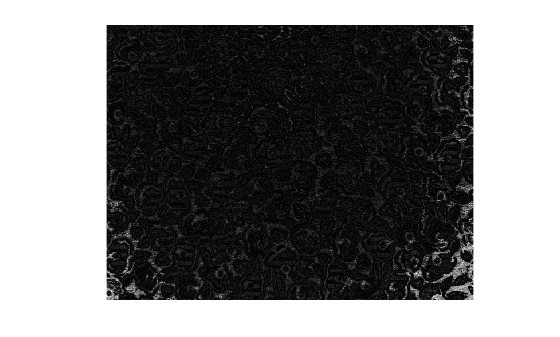## Input Arguments

collapse all

First set of color data, specified in one of these formats.

• 1-by-3 numeric vector representing a reference color.

• c-by-3 numeric matrix representing a set of c colors.

• m-by-n-by-3 numeric array representing a color image.

• Multidimensional numeric array, such as an m-by-n-by-3-by-p array, representing a batch of color images. The third dimension must correspond to the color channel and have 3 channels.

If `I2` is not a reference color, then `I1` must be either a reference color or a numeric array of the same size as `I2`.

`I1` and `I2` must be in the same color space. By default, the `imcolordiff` function interprets the color data as RGB color values. To calculate the color difference in the L*a*b* color space, specify the `isInputLab` argument as `true`. L*a*b* color values can be of data type `single` or `double` only.

Data Types: `single` | `double` | `uint8` | `uint16`

Second set of color data, specified in one of these formats.

• 1-by-3 numeric vector representing a reference color.

• c-by-3 numeric matrix representing a set of c colors.

• m-by-n-by-3 numeric array representing a color image.

• Multidimensional numeric array, such as an m-by-n-by-3-by-p array, representing a batch of color images. The third dimension must correspond to the color channel and have 3 channels.

If `I1` is not a reference color, then `I2` must be either a reference color or a numeric array of the same size as `I1`.

`I1` and `I2` must be in the same color space. By default, the `imcolordiff` function interprets the color data as RGB color values. To calculate the color difference in the L*a*b* color space, specify the `isInputLab` argument as `true`. L*a*b* color values can be of data type `single` or `double` only.

Data Types: `single` | `double` | `uint8` | `uint16`

### Name-Value Arguments

Specify optional pairs of arguments as `Name1=Value1,...,NameN=ValueN`, where `Name` is the argument name and `Value` is the corresponding value. Name-value arguments must appear after other arguments, but the order of the pairs does not matter.

Example: `dE = imcolordiff(I1,I2,Standard="CIEDE2000")` calculates the color difference between two RGB images using the CIEDE2000 standard.

Before R2021a, use commas to separate each name and value, and enclose `Name` in quotes.

Example: `dE = imcolordiff(I1,I2,"Standard","CIEDE2000")` calculates the color difference between two RGB images using the CIEDE2000 standard.

CIE standard used to compute the color difference value, specified as one of these values:

ValueDescription
`"CIE94"`The CIE94 standard. This standard improves the perceptual non-uniformities of the CIE76 standard implemented in the `deltaE` function.
`"CIEDE2000"`The CIEDE2000 standard. This standard further improves the perceptual uniformity through five additional corrections: a hue rotation term, compensation for neutral colors, and compensation for lightness, chroma, and hue.

Data Types: `char` | `string`

Color values are in the L*a*b* color space, specified as a numeric or logical `0` (`false`) or `1` (`true`).

Luminance coefficient, specified as a numeric scalar. `kL` is typically `1` for applications in graphic arts and `2` for applications in textiles.

Chroma compensation coefficient, specified as a numeric scalar. `kC` is typically `1`.

Hue compensation coefficient, specified as a numeric scalar. `kH` is typically `1`.

K1 weighting factor, specified as a numeric scalar. The K1 weighting factor applies to the CIE94 standard only. The value is typically `0.045` for applications in graphic arts and `0.048` for applications in textiles.

K2 weighting factor, specified as a numeric scalar. The K2 weighting factor applies to the CIE94 standard only. The value is typically `0.015` for applications in graphic arts and `0.014` for applications in textiles.

## Output Arguments

collapse all

Color difference (delta E), returned as one of the following.

• An m-by-n numeric matrix. The array represents the pixel-wise color difference between two color images, or between a color image and a reference color.

• A c-by-1 numeric column vector. The vector represents the color difference between two sets of c colors, or between a set of c colors and a reference color.

• A numeric scalar that represents the color difference between two reference colors.

• A multidimensional numeric array. The array represents the pixel-wise color difference between two batches of color images, or between a batch of color images and a reference color. The third dimension has length 1 and indicates the color difference.

If `I1` or `I2` is of data type `double`, then `dE` is of data type `double`. Otherwise, `dE` is of data type `single`.

Data Types: `single` | `double`

## Tips

• To calculate color differences following the CIE76 standard, use the `deltaE` function. This function is faster than the `imcolordiff` function, but less precise.

• Typically, you should keep the default values of the `kL`, `kC`, `kH`, `K1`, and `K2` arguments. The values can change for specific industry groups, such as graphic arts and textiles, based on experimentally validated lighting conditions for that industry.

 Sharma, Gaurav, Wencheng Wu, and Edul N. Dalal, "The CIEDE2000 Color-Difference Formula: Implementation Notes, Supplementary Test Data, and Mathematical Observations." Color Research and Application 30, no. 1 (February 2005): 21–30. https://doi.org/10.1002/col.20070.

 ISO/CIE 11664-6:2014. "Colorimetry — Part 6: CIEDE2000 Colour-difference formula." International Organization for Standardization. URL: https://www.iso.org/standard/63731.html.

 CIE 116-1995. "Industrial Colour-Difference Evaluation (E)." International Commission on Illumination (CIE). URL: https://cie.co.at/publications/industrial-colour-difference-evaluation.